M.M.F. Method of Determining Regulation

This method of determining the regulation of an alternator is also called Ampere-turn method or Rothert's M.M.F. method. The method is based on the results of open circuit test and short circuit test on an alternator.
For any synchronous generator i.e. alternator, it requires m.m.f. which is product of field current and turns of field winding for two separate purposes.
1. It must have an m.m.f. necessary to induce the rated terminal voltage on open circuit.
2. It must have an m.m.f. equal and opposite to that of armature reaction m.m.f.
Note : In most of the cases as number of turns on the field winding is not known, the m.m.f. is calculate and expressed i terms of the field current itself.
The field m.m.f. required to induce the rated terminal voltage on open circuit can be obtained from open circuit test results and open circuit characteristics. This is denoted as FO.
We know that the synchronous impedance has two components, armature resistance and synchronous reactance. Now synchronous reactance also has two components, armature leakage reactance and armature reaction reactance. In short circuit test, field m.m.f. is necessary to overcome drop across armature resistance and leakage reactance and also to overcome effect of armature reaction. But drop across armature resistance and also to overcome effect of armature reaction. But drop across armature resistance and leakage reactance is very small and can be neglected. Thus in short circuit test, field m.m.f. circulates the full load current balancing the armature reaction effect. The value of ampere-turns required to circulate full load current can be obtained from short circuit characteristics. This is denoted as  FAR.
Under short circuit condition as resistance and leakage reactance of armature do not play any significant role, the armature reaction reactance is dominating and hence the power factor of such purely reactive circuit is zero lagging. Hence FAR gives demagnitising ampere turns. Thus the field m.m.f. is entirely used to overcome the armature reaction which is wholly demagntising in nature.
The two components of total field m.m.f. which are FO and FAR are indicated in O.C.C. (open circuit characteristics) and S.C.C. (short circuit characteristics) as shown in the Fig. 1.Fig. 1
If the alternator is supplying full load, then total field m.m.f. is the vector sum of its two components  FO and FAR. This depends on the power factor of the load which alternator is supplying. The resultant field m.m.f. is denoted as FR. Let us consider the various power factors and the resultant FR.
Zero lagging p.f. : As long as power factor is zero lagging, the armature reaction is completely demagnetising. Hence the resultant FR is the algebraic sum of the two components FO and FAR. Field m.m.f. is not only required to produce rated terminal voltage but also required to overcome completely demagnetising armature reaction effect.Fig. 2
This is shown in the Fig. 2.
OA = FO
AB = FAR   demagnetising
OB = FR = FO + FAR
Total field m.m.f. is greater than FO.
Zero leading p.f. : When the power factor is zero leading then the armature reaction is totally magnetising and helps main flux to induce rated terminal voltage. Hence net field m.m.f. required is less than that required to induce rated voltage normally, as part of its function is done by magnetising armature reaction component. The net field m.m.f. is the algebraic difference between the two components FO and FAR. This is shown in the Fig. 3.Fig. 3
OA = FO
AB = FAR magnetising
OB = FO - FAR = FR
Total m.m.f. is less than FO
Unity p.f. : Under unity power factor condition, the armature reaction is cross magnetising and its effect is to distort the main flux. Thus and F are at right angles to each other and hence resultant m.m.f. is the vector sum of FO and FAR. This is shown in the Fig.4.Fig. 4
OA = FO
AB = FAR cross magnetising

General Case : Now consider that the load power factor is cos Φ. In such case, the resultant m.m.f. is to be determined by vector addition of FO and FAR.
cosΦ, lagging p.f. : When the load p.f. is cosΦ lagging, the phase current Iaph lags Vph by angle Φ. The component FO is at right angles to Vph while FAR is in phase with the current Iaph. This is because the armature current Iaph decides the armature reaction. The armature reaction FAR due to current Iaph is to be overcome by field m.m.f. Hence while Finding resultant field m.m.f., - FAR should be added to vectorially. This is because resultant field m.m.f. tries to counterbalance armature reaction to produce rated terminal voltage. The phasor diagram is shown in the Fig. 5.
From the phasor diagram the various magnitude are,
OA = FO ,  AB = FAR , OB = FR
Consider triangle OCB which is right angle triangle. The FAR is split into two parts as,
AC = FAR  sinΦ and BC = FAR cosΦFig. 5

...    ( FR)2 = (FO + FAR  sinΦ )2 + (FAR cosΦ)2                               ................ (1)
From this relation (1), FR can be determined.
cosΦ, leading p.f. : When the load p.f. is cosΦ leading, the phase current Iaph leads Vph by Φ. The component FO is at right angles to Vph and FAR  is in phase with Iaph. The resultant FR can be obtained by adding - FAR  to FO. The phasor diagram is shown in the Fig.6.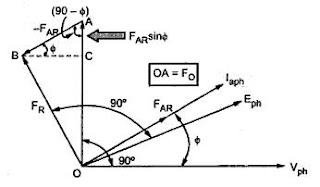Fig. 6
From the phasor diagram, various magnitudes are,
AC = FAR  sinΦ and BC = FAR  cosΦ
OA = FO,   AB = FAR  and OB = FR
Consider triangle OCB which is right angles triangle.
...     (OB)2 = (OC)2 + (BC)2
...    ( FR)2 = (FO - FAR  sinΦ )2 + (FAR cosΦ)                   .................... (2)
From the relation (2), FR can be obtained.
Using relations (1) and (2), resultant field m.m.f. FR for any p.f. load condition can be obtained.
Once FR is known, obtain corresponding voltage which is induced e.m.f. Eph, required to get rated terminal voltage Vph. This is possible from open circuit characteristics drawn.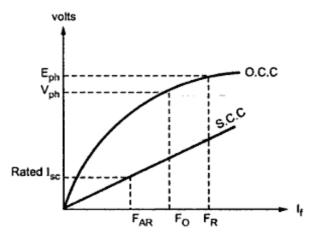Fig. 7
Once Eph is known then the regulation can be obtained as,

Note : To obtain Eph corresponding to FR, O.C.C. must be drawn to the scale, from the open circuit test readings.
Note : This ampere-turn method gives the regulation of an alternator which is lower than the actually observed. Hence the method is called optimistic method.
Important note : When the armature resistance is neglected then FO is field m.m.f. required to produce rated Vph at the output terminals. But if the effective armature resistance is given then FO is to be calculated from O.C.C. such that FO represents the excitation (field current) required a voltage of Vph + Iaph Raph  cosΦ where
Vph = rated voltage per phase
Iaph = full load current per phase
R = armature resistance per phase
cosΦ = power factor of the load
It can also be noted that, FR can be obtained using the cosine rule to the triangle formed by FO, FAR and FO as shown in the Fig. 8.Fig. 8

Using cosine rule to triangle OAB,

Students can use equations 1, 2 or 3 to calculate FR.
The angle between Eo and Vph is denoted as δ and is called power angle. Neglecting R we can write,
Ia Xs cosΦ = Eo sinδ
Pd = Vph Ia cosΦ = internal power of machine

Note : This equation shows that the internal power of the machine is proportional to sin δ.

1.easy to understand ...

2.amp. turns method is optimistic method of determining the voltage regulation of an alternator.

3.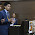This comment has been removed by the author.

4.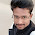how to calculate Eph

5.why is voltage regulation less than actual voltage regulation

6.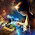Enough for the topic to understand.